# Python – Tkinter askquestion Dialog

In Python, There Are Several Libraries for Graphical User Interface. Tkinter is one of them that is most useful. It is a standard interface. Tkinter is easy to use and provides several functions for building efficient applications. In Every Application, we need some Message to Display like “Do You Want To Close ” or showing any warning or Something information. For this Tkinter provide a library like messagebox. By using the message box library we can show several Information, Error, Warning, Cancellation ETC in the form of Message-Box. It has a Different message box for a different purpose.

1. showinfo() – To display some important information .
2. showwarning() – To display some type of Warning.
3. showerror() –To display some Error Message.
4. askquestion() – To display a dialog box that asks with two options YES or NO.
5. askokcancel() – To display a dialog box that asks with two options OK or CANCEL.
6. askretrycancel() – To display a dialog box that asks with two options RETRY or CANCEL.
7. askyesnocancel() – To display a dialog box that asks with three options YES or NO or CANCEL.

Syntax of the Message-Box Functions:

`  messagebox.name_of_function(Title, Message, [, options])`
1. name_of_function – Function name that which we want to use .
2. Title – Message Box’s Title.
3. Message – Message that you want to show in the dialog.
4. Options –To configure the options.

This function is used to ask questions to the user. That has only two options YES or NO.
Application of this function:

1. We can use this to ask the user if the User want’s to continue.
2. We can use this to ask the user if the User wanted to Submit or not.

Syntax:

`messagebox.askfunction((Title, Message, [, options])`

Example:

## Python3

 `from` `tkinter ``import` `*` `from` `tkinter ``import` `messagebox` ` `  `    `  `# object of TK()` `main ``=` `Tk()`   `# function to use the ` `# askquestion() function` `def` `Submit(): ` `    ``messagebox.askquestion(``"Form"``,` `                           ``"Do you want to Submit"``)` `    `  `# setting geometry of window ` `# instance` `main.geometry(``"100x100"``)`   `# creating Window` `B1 ``=` `Button(main, text ``=` `"Submit"``, command ``=` `Submit) `   `# Button positioning ` `B1.pack()   `   `# infinite loop till close` `main.mainloop()  `

Output: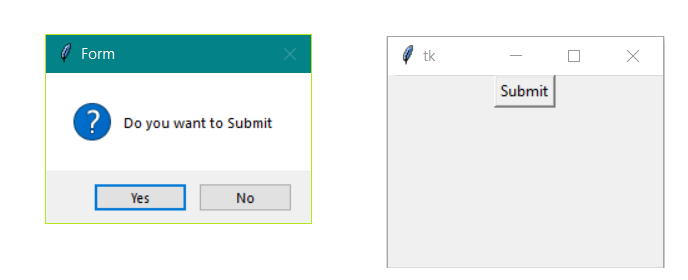1. Importing the Libraries
To use the GUI functionality in python we have to import the libraries. In the first line, we are importing Tkinter, and second-line we’re importing messagebox library

```from tkinter import *
from tkinter import messagebox```

2. Main window instance
We have to create an instance or object for the window to TK(); Tk() is a function of Tkinter that create a window that can be referred from the main variable

`main = Tk()  `

3. Set dimension
we set the dimension of the window we can set it in various ways.in this we are setting is by geometry() function of size “100X100”.

`top.geometry("100x100")  `

4. Applying other widget and function
In our example, we create a method named Submit and call the askquestion() and Creating Button and setting it by Pack() function

```def Submit():
messagebox.askquestion("Form", "Do you want to Submit")

main.geometry("100x100")
B1 = Button(main, text = "Submit", command = Submit)
B1.pack()  ```

5. mainloop()
This method can be used when all the code is ready to execute.It runs the INFINITE Loop used to run the application. A window will open until the close button is pressed.

ICONS that We can use in Options

1. Error
2. Info
3. Warning
4. Question

We can change the icon of the dialog box. The type of icon that we want to use just depends on the application’s need. we have four icons.

Error

` messagebox.function_name(Title, Message, icon='error')`

Example-

## Python3

 `# illustration of icon - Error` `from` `tkinter ``import` `*` `from` `tkinter ``import` `messagebox`   `main ``=` `Tk()`   `def` `check():` `   ``messagebox.askquestion(``"Form"``, ` `                          ``"Is your name correct?"``, ` `                          ``icon ``=``'error'``)`   `main.geometry(``"100x100"``)` `B1 ``=` `Button(main, text ``=` `"check"``, command ``=` `check)` `B1.pack()`   `main.mainloop()`

Output: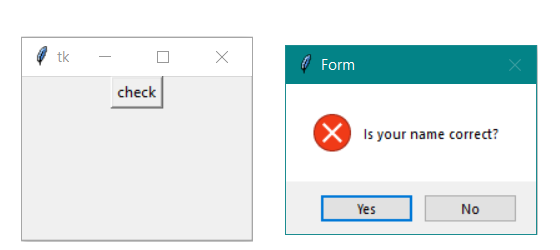info

` messagebox.function_name(Title, Message, icon='info')`

Example-

## Python3

 `# illustration of icon - Info` `from` `tkinter ``import` `*` `from` `tkinter ``import` `messagebox`   `main ``=` `Tk()`   `def` `check():` `   ``messagebox.askquestion(``"Form"``,` `                          ``"do you want to continue"``, ` `                          ``icon ``=``'info'``)`   `main.geometry(``"100x100"``)` `B1 ``=` `Button(main, text ``=` `"check"``, command ``=` `check)` `B1.pack()`   `main.mainloop()`

Output: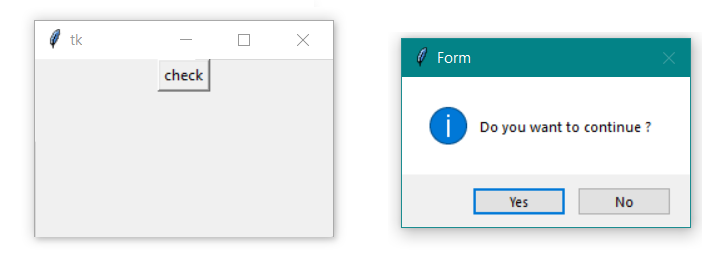Question

` messagebox.function_name(Title, Message, icon='question')`

Example-

## Python3

 `# illustration of icon - question` `from` `tkinter ``import` `*` `from` `tkinter ``import` `messagebox`   `main ``=` `Tk()`   `def` `check():` `   ``messagebox.askquestion(``"Form"``,` `                          ``"are you 18+"``, ` `                          ``icon ``=``'question'``)`   `main.geometry(``"100x100"``)` `B1 ``=` `Button(main, text ``=` `"check"``, command ``=` `check)` `B1.pack()`   `main.mainloop()`

Output: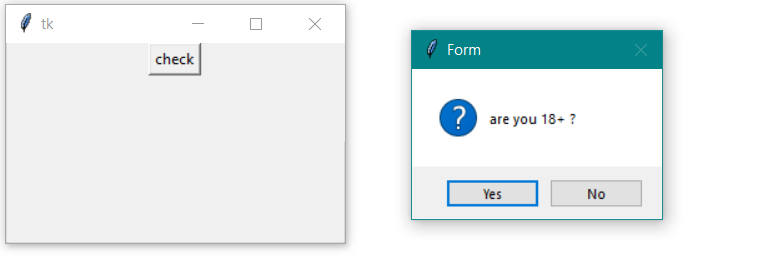Warning

`messagebox.function_name(Title, Message, icon='warning')`

Example-

## Python3

 `# illustration of icon - Warning` `from` `tkinter ``import` `*` `from` `tkinter ``import` `messagebox`   `main ``=` `Tk()`   `def` `check():` `   ``messagebox.askquestion(``"Form"``,` `                          ``"Gender is empty?"``,` `                          ``icon ``=``'warning'``)`   `main.geometry(``"100x100"``)` `B1 ``=` `Button(main, text ``=` `"check"``, command ``=` `check)` `B1.pack()`   `main.mainloop()`

Output: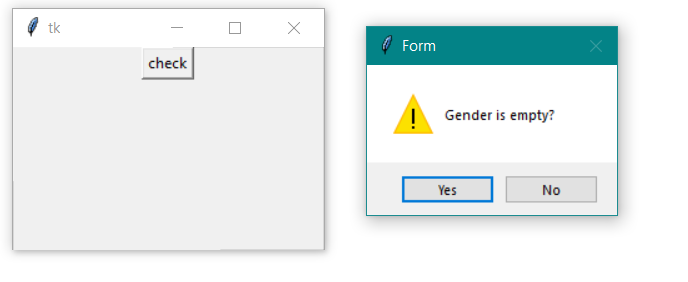Whether you're preparing for your first job interview or aiming to upskill in this ever-evolving tech landscape, GeeksforGeeks Courses are your key to success. We provide top-quality content at affordable prices, all geared towards accelerating your growth in a time-bound manner. Join the millions we've already empowered, and we're here to do the same for you. Don't miss out - check it out now!

Previous
Next